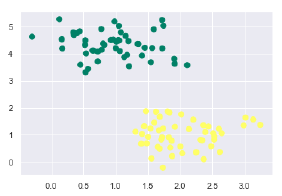## Support Vector Machine Learning:

Support Vector Machine Learning is most important topic in machine learning so friends today Let's start with SVM in Machine Learning.

### Overview ON SVM in machine learning

1. What is support vector machine (SVM)
2. Implementation of SVM in Python
3. SVM different applications
4. Example on SVM
Think of machine learning algorithms as an armoury packed with axes, sword, blades, bow, dagger, etc. You have various tools, but you ought to learn to use them at the right time. As an analogy, think of Regression as sword capable of slicing and dicing data efficiently, but incapable of dealing with highly complex data. On the contrary, ‘Support Vector Machines’ is like a sharp knife – it works on smaller datasets, but on the complex ones, it can be much stronger and powerful in building machine learning models.

### Application of  SVM in Machine Learning:

1.Identify the right hyper-plane
2.Can we classify two classes
3.Find the hyper-plane to segregate to classes

### Example on Support Vector Machine Learning :

For implementing Support Vector Machine in Python we will start with the standard libraries import as follows −

```
import numpy as np

import matplotlib.pyplot as plt
from scipy import stats

import seaborn as sns; sns.set()
```

Next, we are creating a sample dataset, having linearly separable data, from sklearn.dataset.sample_generator for classification using Support Vector Machine−

```
from sklearn.datasets.samples_generator import make_blobs

X, y = make_blobs(n_samples = 100, centers = 2, random_state = 0, cluster_std = 0.60)

plt.scatter(X[:, 0], X[:, 1], c = y, s = 60, cmap = 'summer');
```

OUTPUT:Tags:Support Vector Machine Learning-SVM-algorithms-python
BEST OF LUCK!!!

Previous
Next Post »

## Featured Snippet

### 10 ways to iterate through a list in python

10 ways to iterate through a list in python The list is similar to array in other languages except for python, which provides the extra b...